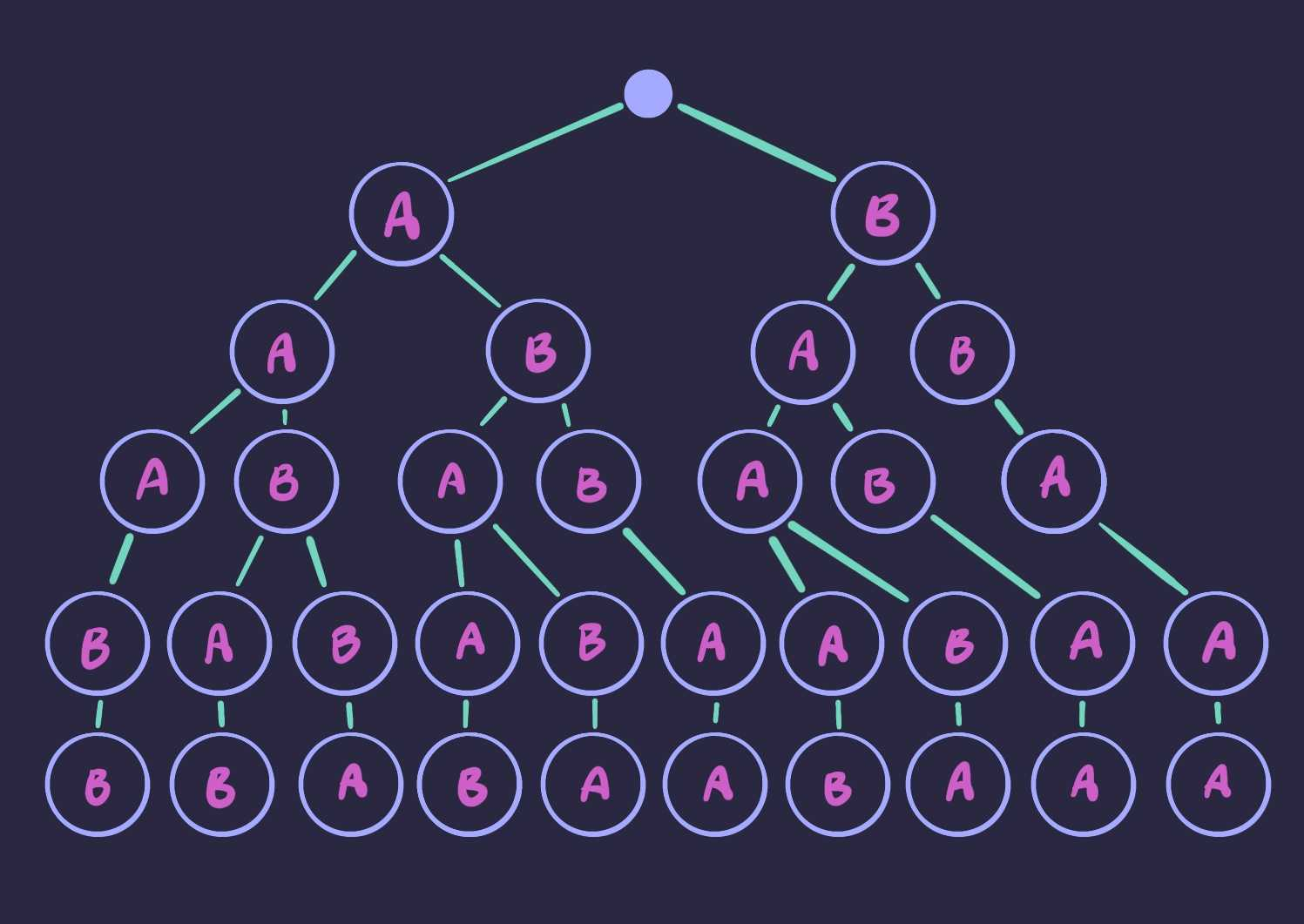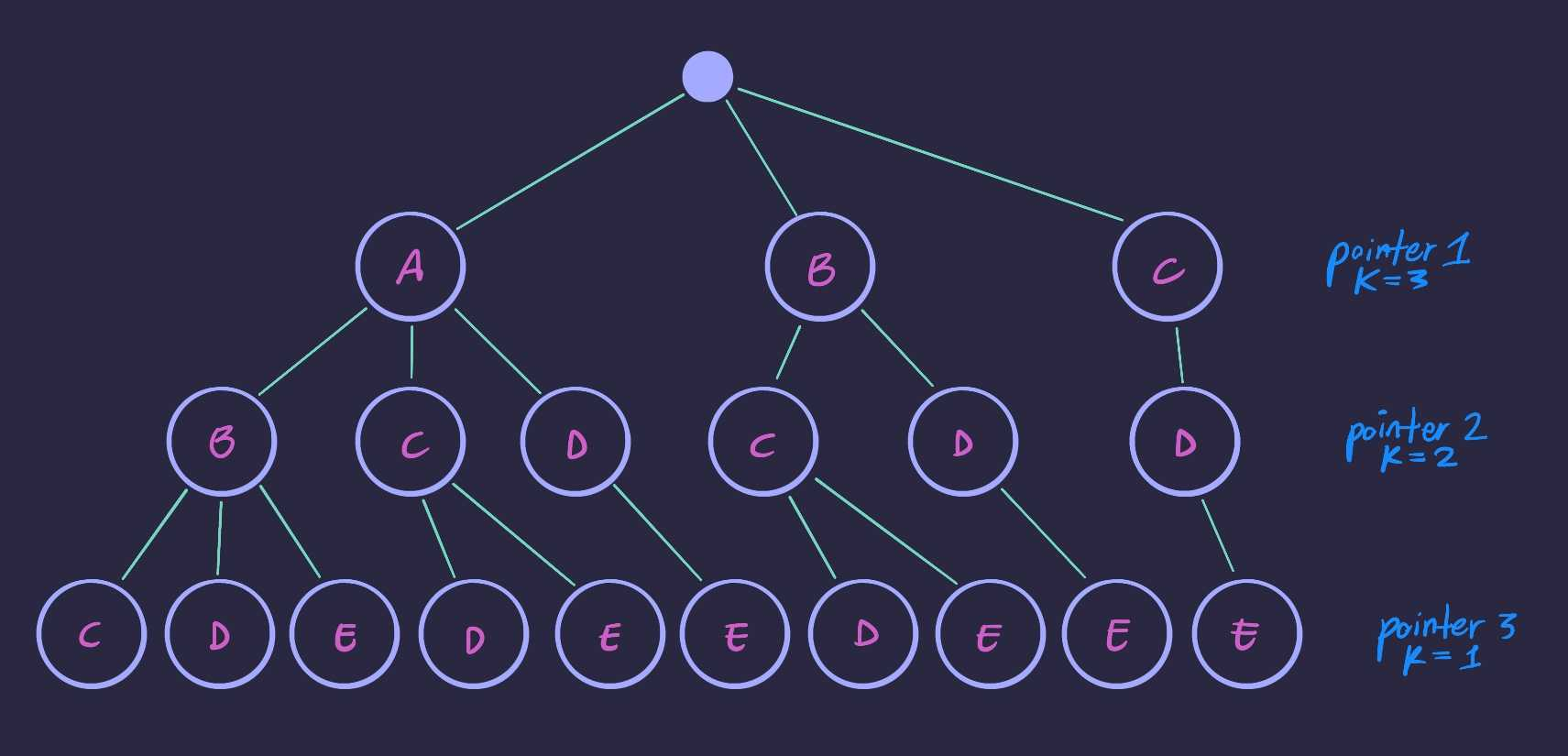# Math & Code: Permutations and Combinations

Jun. 07, 2020
905 words

## Permutations### Generate all Permutations

How many ways can you arrange the characters A, B, C?

Choose any character to start. Then from the remaining two, choose one. Then from the remaining one, choose it.

There are $n!$ ways to do this. So we have $3! = 3*2*1 = 6$.

We can get all the permutations by generating the following tree. Each branching point represents a decision. Each complete path from root to leaf represents a permutation:def print_perms(perm: list, options: list) -> None:
if not options:
print(perm)
for i, item in enumerate(options):
print_perms(perm+[item], options[:i]+options[i+1:])
>>> print_perms([], ['A', 'B', 'C'])

>>> ['A', 'B', 'C']
>>> ['A', 'C', 'B']
>>> ['B', 'A', 'C']
>>> ['B', 'C', 'A']
>>> ['C', 'A', 'B']
>>> ['C', 'B', 'A']

### Permutations with Repeat Characters

How many unique permutations are there for A, A, A, B, B, C?

We have six slots _ _ _ _ _ _ in which to place characters. Let’s place the A’s first. We need to choose three of the available six slots. There’s $\binom{6}{3}$ ways to do this.

Suppose we came up with A _ A A _ _. Now place the B characters. Three spots left for two chars, so $\binom{3}{2}$ ways to do this.

Now maybe we have A B A A _ B. There’s $\binom{1}{1}$ ways to place the C.

$\binom{6}{3} \binom{3}{2} \binom{1}{1} = 20*3*1 = 60$

There are 60 unique permutations for this string. If all 6 characters were unique there would be $6! = 720$ permutations, so the duplication has a big effect.

There is another way to calculate this. Count all permutations as-if all characters were unique. Now divide by the number of ways you could permute each bunch of characters (to fix the over-counting).

There’s three A characters, so $3!$. Two B characters: $2!$. And one C, $1!$.

$\frac{6!}{(3!)(2!)(1!)} = 60$

We can modify the code from above to deal with duplicate characters properly.

def print_perms(perm: list, options: list) -> None:
if not options:
print(perm)
for i, item in enumerate(set(options)):
new_options = options[:]
new_options.remove(item)
print_perms(perm+[item], new_options)
>>> print_perms([], ['A', 'A', 'A', 'B', 'B'])

>>> ['B', 'B', 'A', 'A', 'A']
>>> ['B', 'A', 'B', 'A', 'A']
>>> ['B', 'A', 'A', 'B', 'A']
>>> ['B', 'A', 'A', 'A', 'B']
>>> ['A', 'B', 'B', 'A', 'A']
>>> ['A', 'B', 'A', 'B', 'A']
>>> ['A', 'B', 'A', 'A', 'B']
>>> ['A', 'A', 'B', 'B', 'A']
>>> ['A', 'A', 'B', 'A', 'B']
>>> ['A', 'A', 'A', 'B', 'B']

Now we’re iterating over the set of options (so no duplicates) at each step. We still pass down the full list once we make our branching decision (minus the option taken). This must be done in order to keep track of how instances of each character are remaining.## Combinations### Generate all subsets

How many subsets can be made from the set $\{A, B, C\}$?

All valid subsets for this example will have size $0$, $1$, $2$, or $3$. If we can figure out how many subsets exist for each size, we can sum those to the answer.

The number of ways you can make a subset of size $k$ given a set of size $n$ is $\binom{n}{k}$. So we can just sum $\binom{n}{k}$ where $n$ is the size of the set, and where $k$ goes from $0$ to $n$. Summing those we get:

$\sum_{k=0}^{n}\binom{n}{k} = 2^n$

One way to make sense of the $2^n$ result is to think that, for a given subset, every element from the parent set will either be present, or not. So, every subset can be represented by a binary string:

{A, B, C}
[0, 0, 0] -> {}
[0, 0, 1] -> {C}
[0, 1, 0] -> {B}
[0, 1, 1] -> {B, C}
[1, 0, 0] -> {A}
[1, 0, 1] -> {A, C}
[1, 1, 0] -> {A, B}
[1, 1, 1] -> {A, B, C}

We can also use this mapping scheme, from binary string to subset, to generate all $2^n$ subsets.

def subsets(s: list) -> None:
n = len(s)
# count up from 0 to 2^n
for i in range(2**n):
# get the bit representation of i, convert to list
bits = list(f'{i:0b}')
bits = ['0']*(n-len(bits)) + bits
# include element in subset if bit vector says to
print([s[j] for j in range(n) if bits[j] == '1'])
>>> subsets(['A', 'B', 'C'])

>>> []
>>> ['C']
>>> ['B']
>>> ['B', 'C']
>>> ['A']
>>> ['A', 'C']
>>> ['A', 'B']
>>> ['A', 'B', 'C']

### Generate all subsets of size k

What if we only want to generate subsets of size $k$?

There’s $\binom{n}{k}$ ways to do this. We could just generate every subset and filter out the ones we don’t want, but that would be bad.

Suppose $k=3$, we could do something like this:

['A', 'B', 'C', 'D', 'E']
^    ^    ^
['A', 'B', 'C', 'D', 'E']
^    ^         ^
['A', 'B', 'C', 'D', 'E']
^    ^              ^
['A', 'B', 'C', 'D', 'E']
^         ^    ^
['A', 'B', 'C', 'D', 'E']
^         ^         ^
['A', 'B', 'C', 'D', 'E']
^              ^    ^
['A', 'B', 'C', 'D', 'E']
^    ^    ^
['A', 'B', 'C', 'D', 'E']
^    ^         ^
['A', 'B', 'C', 'D', 'E']
^         ^    ^
['A', 'B', 'C', 'D', 'E']
^    ^    ^

When the last pointer goes as far right as it can, advance the second-to-last pointer one spot, and pull the last pointer back close to it. When the second-to-last pointer goes as far right as it can, advance the third-to-last pointer one spot, and pull back all pointers on its right side close to it. Repeat this process until all pointers are bunched up on the right side.

This iteration scheme can be implemented recursively:

def subsets_size_k(subset: list, options: list, k: int) -> None:
# we're on the last/right-most pointer
# so print the current subset/path plus each option remaining
if k == 1:
for option in options:
print(subset + [option])

# pointer can only move right until it runs into the others
for i in range(len(options[:-(k - 1)])):
# pass down current subset plus path chosen,
# the portion of array to the right of the pointer,
# and decrement k
subsets_size_k(subset+[options[i]], options[i+1:], k-1)
>>> subsets_size_k([], ['A', 'B', 'C', 'D', 'E'], 3)

>>> ['A', 'B', 'C']
>>> ['A', 'B', 'D']
>>> ['A', 'B', 'E']
>>> ['A', 'C', 'D']
>>> ['A', 'C', 'E']
>>> ['A', 'D', 'E']
>>> ['B', 'C', 'D']
>>> ['B', 'C', 'E']
>>> ['B', 'D', 'E']
>>> ['C', 'D', 'E']

This code is effectively building the following tree:### Climbing Stairs

From Climbing Stairs on leetcode:

You are climbing a stair case. It takes $n$ steps to reach to the top. Each time you can either climb 1 or 2 steps. In how many distinct ways can you climb to the top?

The problem can be framed a bit differently as,

how many ways can you arrange the numbers 1 and 2 to sum to $n$?

If $n = 4$ there are 5 valid sequences:

(1, 1, 1, 1) # zero 2's
(2, 1, 1), (1, 2, 1), (1, 1, 2) # one 2
(2, 2) # two 2's

If $n = 5$ there are 8 valid sequences:

(1, 1, 1, 1, 1) # zero 2's
(2, 1, 1, 1), (1, 2, 1, 1), (1, 1, 2, 1), (1, 1, 1, 2) # one 2
(2, 2, 1), (2, 1, 2), (1, 2, 2) # two 2's

For each row you have some number of 2’s that can be placed into a fixed number of “slots”.

On the second row above, there is a single 2 that you can place in any of 4 possible positions. Another way to phrase this is that you have 4 options, and you can choose one – you can choose one of the spots for your 2. The number of ways to do this is $\binom{n}{k}$, where $n$ is the number of slots, and $k$ is the number of 2’s that you are placing.

$\binom{n}{k} = \frac{n!}{k!(n-k)!}$

On each row the values for $n$ and $k$ change though. For each row we add a 2, and remove a 1, so $n$ decrements and $k$ increments. If we calculate $\binom{n}{k}$ for each row and sum them all up, we get the answer.

import math

def climbStairs(n: int) -> int:
sum = 0
k = 0
while n >= k:
sum += math.factorial(n) \
// math.factorial(k) \
// math.factorial(n - k)
n -= 1
k += 1

return sum

This approach can be made quite a bit faster with memoization. Not only do we compute the factorial of some values multiple times, but multiple calls to the factorial function repeats a lot of multiplication.

We will need the factorials for $0$ to $n$, so we can just pre-compute them. We can also use each entry in the table to find the next value. This way no operations are duplicated.

def climbStairs(n: int) -> int:
factorials =  * (n + 1)
for i in range(1, n + 1):
factorials[i] = factorials[i - 1] * i

sum = 0
k = 0
while n >= k:
sum += factorials[n] // factorials[k] // factorials[n - k]
n -= 1
k += 1

return sum